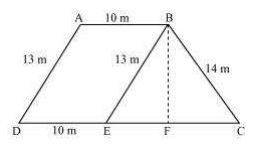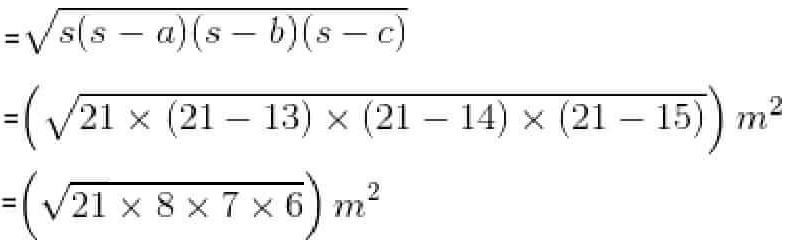Guru

# A field is in the shape of a trapezium whose parallel sides are 25 m and 10 m. The non-parallel sides are 14 m and 13 m. Find the area of the field. Q.9

• 0

What is the easiest way for solving the question of class 9th ncert math of exercise 12.2  of math of Heron’s Formula chapter of question no.9  Please help me for the best suggestion of this question A field is in the shape of a trapezium whose parallel sides are 25 m and 10 m. The non-parallel sides are 14 m and 13 m. Find the area of the field.

Share

1. First, draw a line segment BE parallel to the line AD. Then, from B, draw a perpendicular on the line segment CD.Now, it can be seen that the quadrilateral ABED is a parallelogram. So,

AB = ED = 10 m

AD = BE = 13 m

EC = 25-ED = 25-10 = 15 m

Now, consider the triangle BEC,

Its semi perimeter (s) = (13+14+15)/2 = 21 m

By using Heron’s formula,

Area of ΔBEC == 84 m2

We also know that the area of ΔBEC = (½)×CE×BF

84 cm2 = (½)×15×BF

BF = (168/15) cm = 11.2 cm

So, the total area of ABED will be BF×DE i.e. 11.2×10 = 112 m2

∴ Area of the field = 84+112 = 196 m2

• 0